Question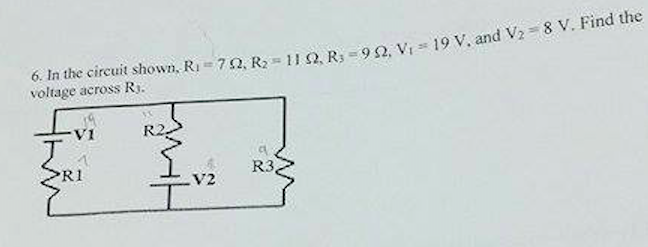Here ,

the current in resistor R1 is I1

current in resistor is R2 is I2 ,

using KVL

19 - 7 * I1 - 9 *(I1 - I2) = 0

16 I1 - 9 I2 = 19 ----(1)

again using KVL

- 11 * I2 + 8 - 9 (I2 - I1) = 0

-9 I1 + 20 I2 = 8    ----(2)

solving 1 and 2

I1 = 1.89 A

I2 = 1.25 A

Now,

V(R3) = (1.89 - 1.25) * 9

V(R3) = 5.76 V

voltage across R3 is 5.76 V

#### Earn Coins

Coins can be redeemed for fabulous gifts.

Similar Homework Help Questions
• ### In the circuit, V1=129 V, V2=72 V, I0=2 A, R1=7 Ohm, R2=46 Ohm, R3=19 Ohm, R4=19...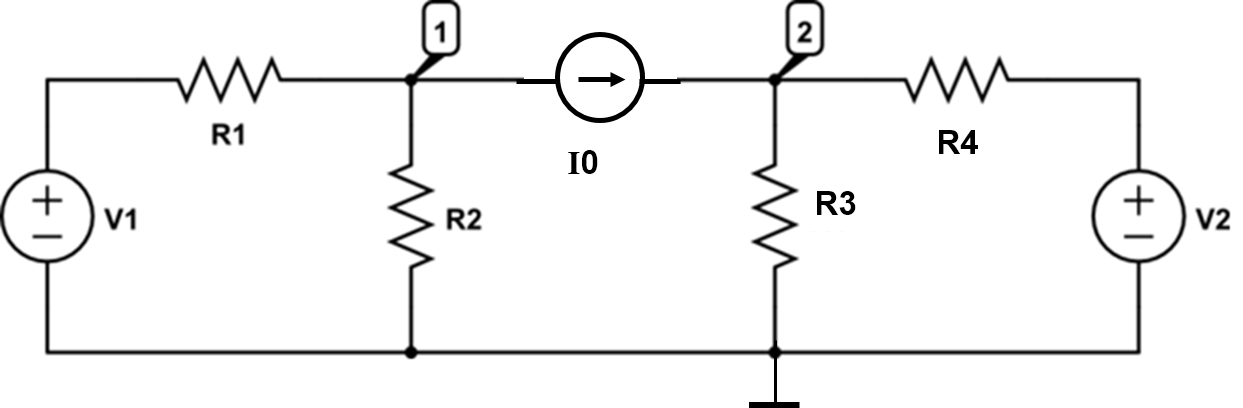In the circuit, V1=129 V, V2=72 V, I0=2 A, R1=7 Ohm, R2=46 Ohm, R3=19 Ohm, R4=19 Ohm. Solve the circuit using nodal analysis and answer the questions below. Find the current through R1 flowing in the direction from left to right. (Unit: A) Find the current through R2 flowing downwards. (Unit: A) Find the current through R3 flowing downwards. (Unit: A) Find the current thrrough R4 flowing from left to right. (Unit: A) R1 R4

• ### pls help R1 R3 R2 w w V14 V3+ V2+ Consider the circuit shown below, where...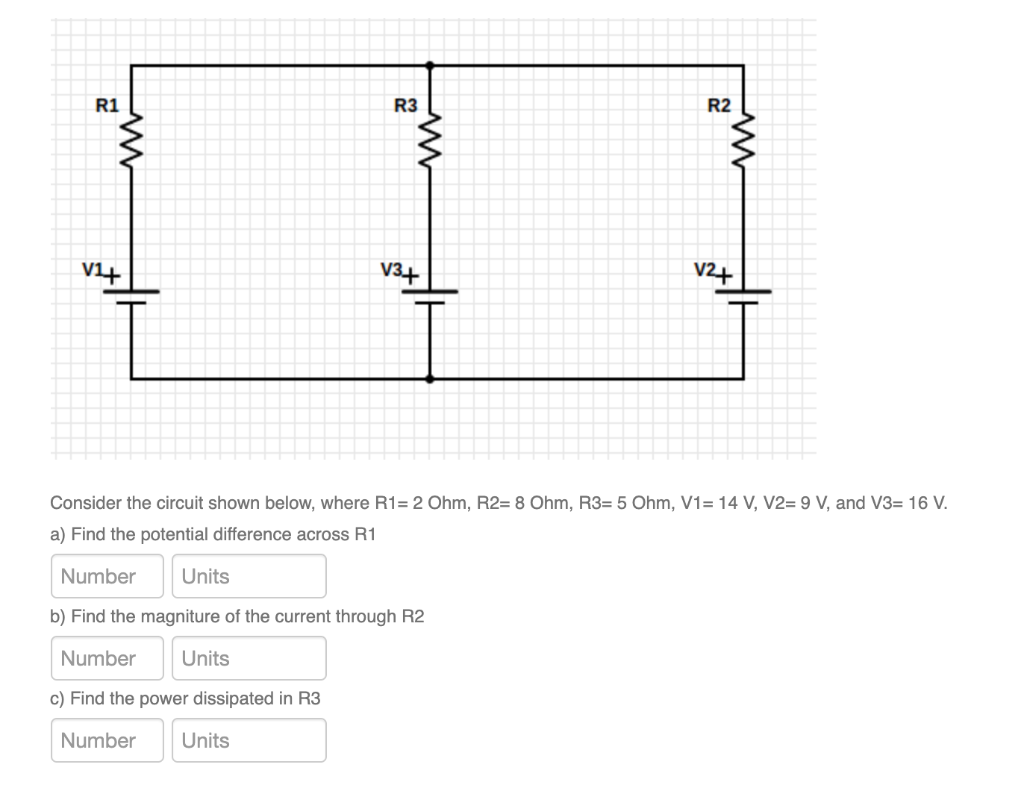pls help R1 R3 R2 w w V14 V3+ V2+ Consider the circuit shown below, where R1= 2 Ohm, R2= 8 Ohm, R3= 5 Ohm, V1= 14 V, V2= 9 V, and V3= 16 V. a) Find the potential difference across R1 Number Units b) Find the magniture of the current through R2 Number Units c) Find the power dissipated in R3 Number Units

• ### For the circuit shown in the figure, If R1=512, R2=10N, R3=3N, V1= V1}V, V2=5V and V3=7V....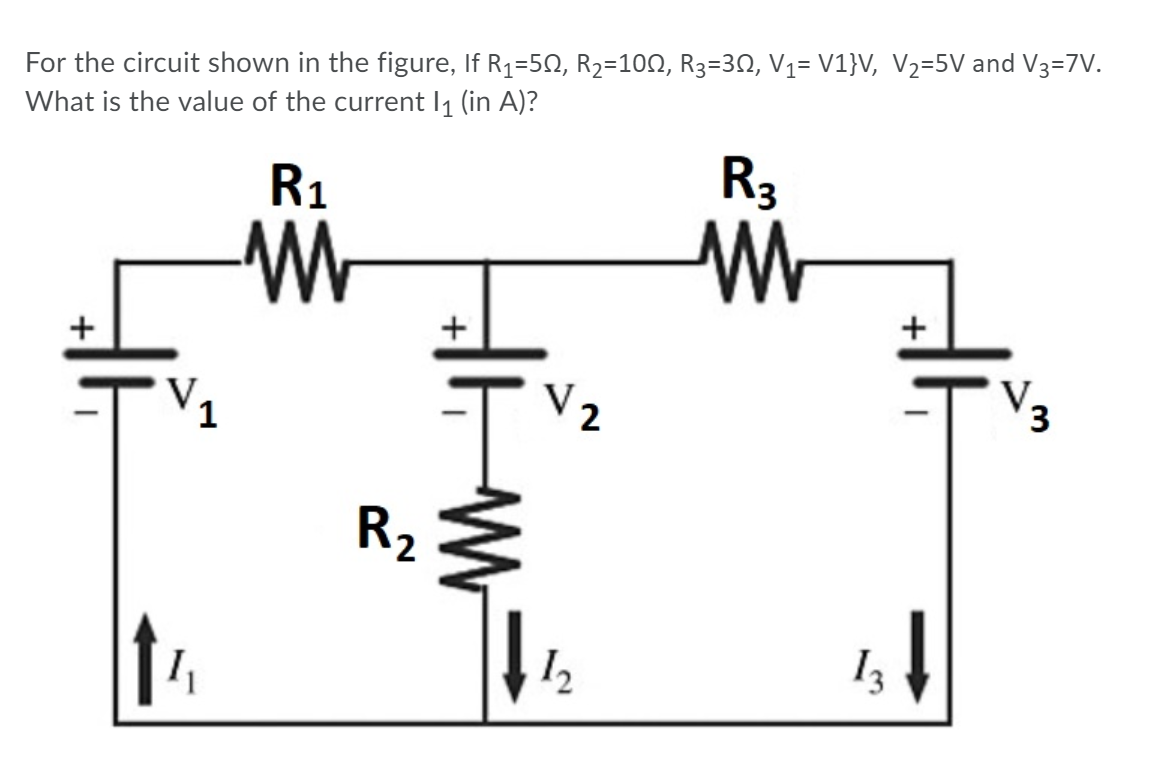For the circuit shown in the figure, If R1=512, R2=10N, R3=3N, V1= V1}V, V2=5V and V3=7V. What is the value of the current 11 (in A)? R1 -W R3 M + + V2 V. 3 R2 \$ 12 13

• ### Consider the circuit shown in the figure with resistors R1= 4.0 Ω, R2= 3.0 Ω, R3=...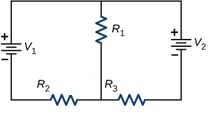Consider the circuit shown in the figure with resistors R1= 4.0 Ω, R2= 3.0 Ω, R3= 3.0 Ω, and batteries V1= 2.0 V, V2=1.5 V respectively. What is the potential drop (voltage) across resistor R3?

• ### Consider the circuit shown below: R1 = 600 ohm R2 = 480 ohm R3 = 240...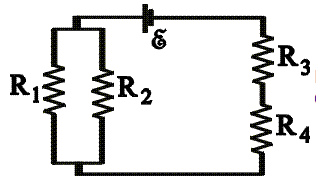Consider the circuit shown below: R1 = 600 ohm R2 = 480 ohm R3 = 240 ohm R4 = 210 ohm ε = 4.5 V a)Calculate the potential difference across R4. b)Calculate the power dissipated in R3. AA CN.

• ### R1 13 |12 Vg V2 R3 R2 18 Ohm and R3 16 Ohm. 90 V, R2...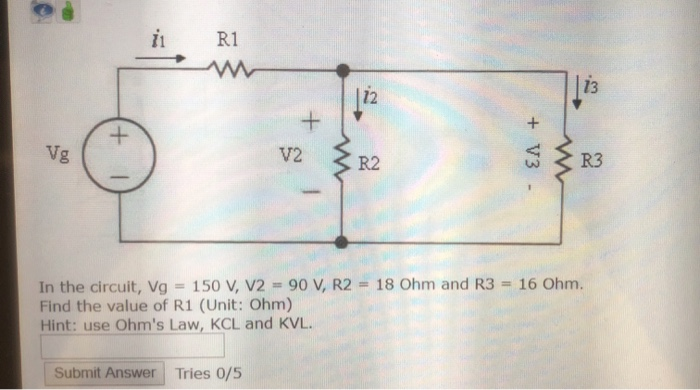R1 13 |12 Vg V2 R3 R2 18 Ohm and R3 16 Ohm. 90 V, R2 In the circuit, Vg Find the value of R1 (Unit: Ohm) Hint: use Ohm's Law, KCL and KVL 150 V, V2 Submit Answer Tries 0/5 +V3 +

• ### In the circuit shown in the figure. R1 = 15,00 Ohm, R2 = 10.0 Ohm, R3...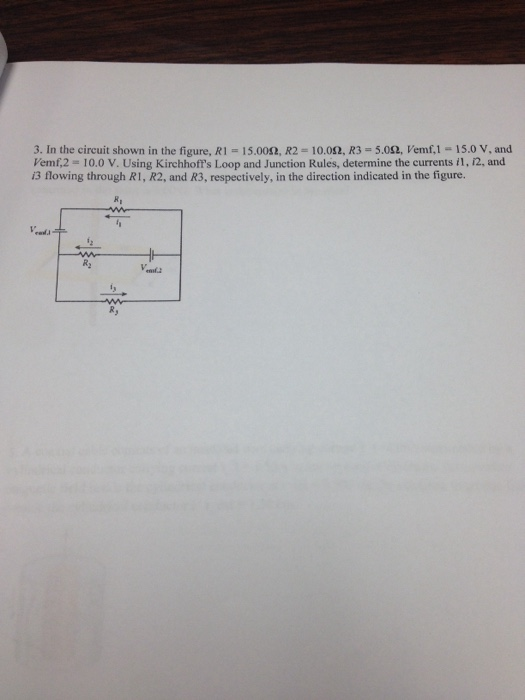In the circuit shown in the figure. R1 = 15,00 Ohm, R2 = 10.0 Ohm, R3 = 5.0 Ohm, V emf,1 = 15.0 V. and Vemf,2 = 10.0 V. Using Kirchhoff's Loop and Junction Rules, determine the currents i1, i2, and i3 flowing through R1, R2, and R3, respectively, in the direction indicated in the figure.

• ### In the circuit below given that R1 = 500 Ohm, R2 = 820 Ohm, R3 =...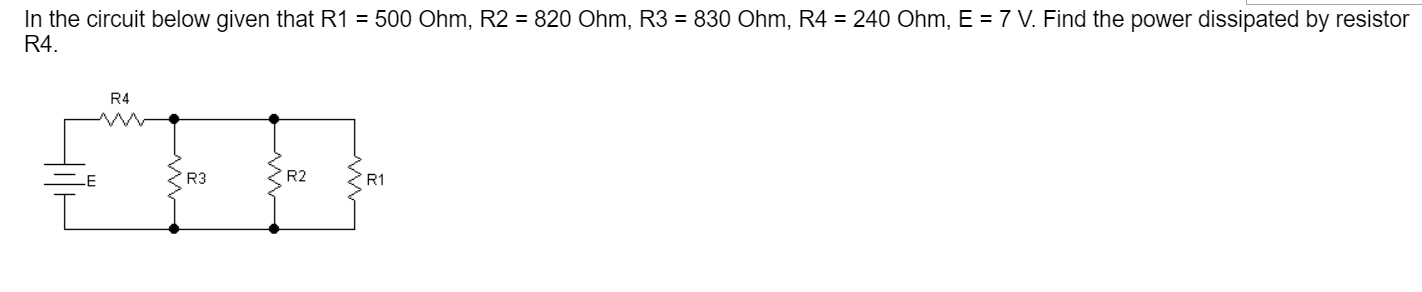In the circuit below given that R1 = 500 Ohm, R2 = 820 Ohm, R3 = 830 Ohm, R4 = 240 Ohm, E = 7 V. Find the power dissipated by resistor R4. R4 E R3 R2 R1

• ### R1 C1 HH The V1 R2 11 R3 circuit shown is a simplified representation of a...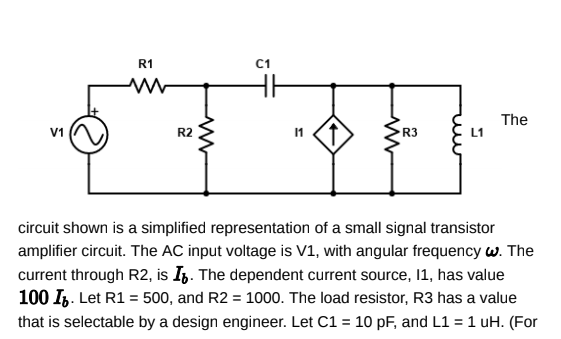R1 C1 HH The V1 R2 11 R3 circuit shown is a simplified representation of a small signal transistor amplifier circuit. The AC input voltage is V1, with angular frequency w. The current through R2, is It. The dependent current source, 11, has value 100 IV. Let R1 = 500, and R2 = 1000. The load resistor, R3 has a value that is selectable by a design engineer. Let C1 = 10 pF, and L1 = 1 uH. (For reference,...

• ### 3) For the circuit shown in the figure, R1 = 180, R2 = 44Q, R3 =...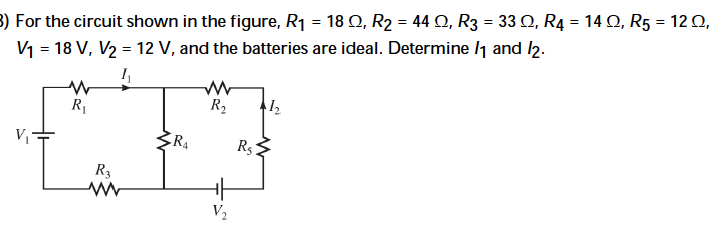3) For the circuit shown in the figure, R1 = 180, R2 = 44Q, R3 = 330, R4 = 140, R5 = 120, V1 = 18 V, V2 = 12 V, and the batteries are ideal. Determine 11 and 12. R R₂ 12 v ZR4 R3